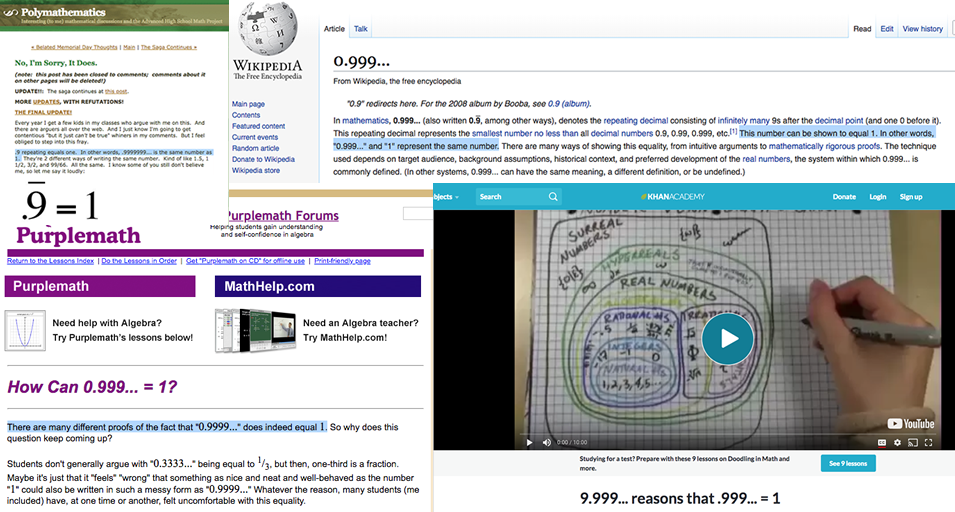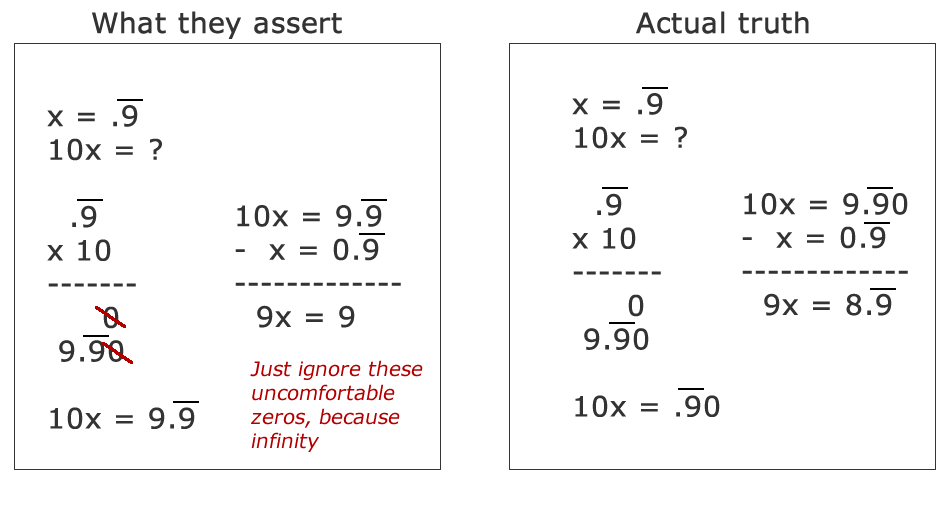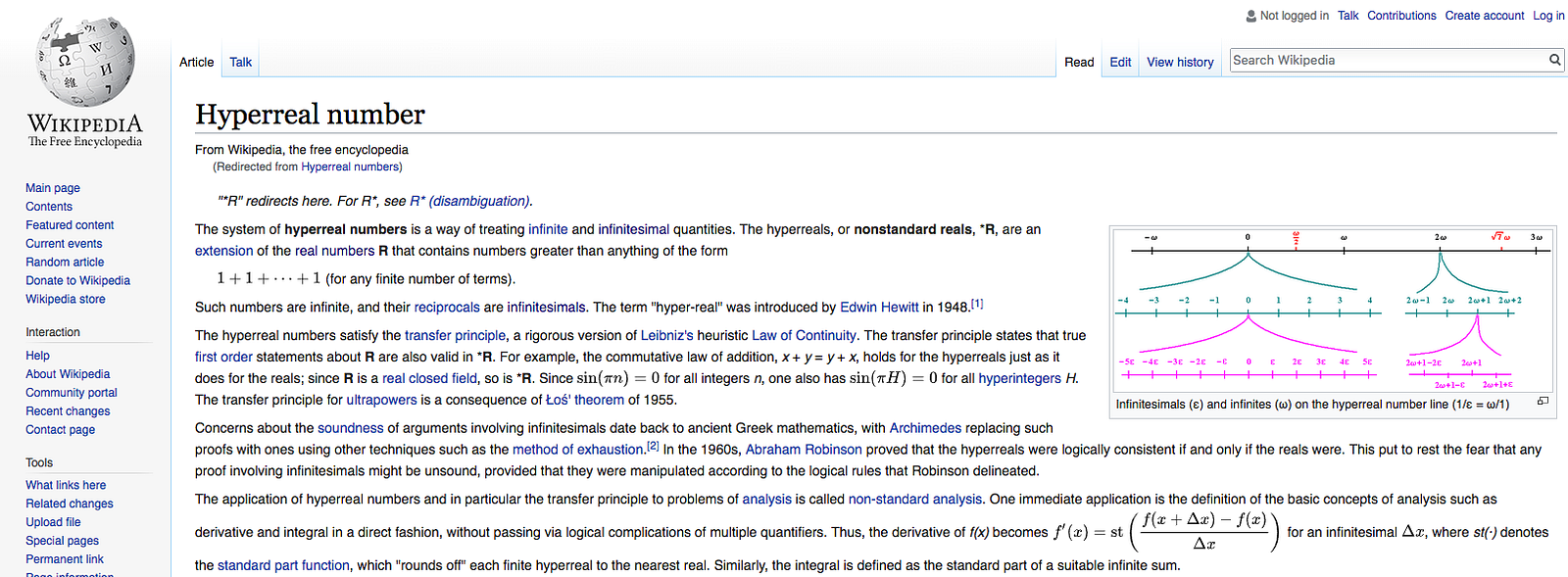# Proof that .99999… is NOT equal to 1

Several years ago, while traveling with co-workers, the subject of .99999… ( that’s .9 with a bar over it, or .9 repeating infinitely ) being equal to 1 came up. While the assertion initially sounds absurd, a quick search on the internet will turn up a plethora of “proofs” and explanations why such an assertion is true. It seems that .99999… really does = 1. That is, at least, until one applies true analytical rigor to the proofs and finds that they are all fallacious.Images from Polymathematics, Wikipedia, Purplemath, and Khan Academy. And they’re all wrong.

It’s difficult to prove that everyone else ( including experts in the field ) is wrong and that some amateur with an argumentative streak ( me ) is right. And it should be. As the individual with a contrary viewpoint, the burden of proof should lie on me. To prove that .99999… is not equal to 1, I will first dismantle the common “proofs” that .99999… = 1. Then, because disproving something does not automatically make the alternately proposed answer true, I will prove my own assertion, that .99999… != 1.

#### Everyone agrees that 1/3 = .33333…

One of the most simplistic arguments that .99999… = 1 is that 1/3 = .33333… and if you multiply both by 3, you get the answer that 3/3 = .99999… Since 3/3 is obviously 1, .99999… must therefore also = 1. The problem here is, 1/3 is not perfectly equal to .33333… Even my early-school math teachers knew that fact. When first learning how to convert fractions to decimals, or decimals to fractions, we are told that .33333… is the “best approximation possible” for 1/3, not that they are actually equal numbers. Don’t believe me? Try to convert .33333… into 1/3. You can create 3/10, 33/100, 333/1000, 3333/10000, etc … but you can never create 1/3. In order for two numbers to be exactly equal to each other, you must be able to convert its visual representation both ways. The truth is, .33333… is just a ‘lazy’ approximation of 1/3. The two numbers are not actually equal.

#### If x = .99999…, then 10x = 9.99999… and 9x = 9, so x = 1

This proof of 10x -x is especially insidious, as it is a simple case of algebraic trickery. The trick behind this proof is that the troublesome part of the number, the infinitely repeating part, is simply removed by subtraction. We have extracted a finite portion of an infinitely repeating number through multiplication and algebraic trickery.

Because the refutation of this proof can be somewhat abstract and esoteric, let me approach it from a different angle. Show to me any finite representation of .99999… in which that number subtracted from 1 gives a non-zero answer. If you say to me that we cannot take a finite representation of an infinite number, then you have disproven 10x -x because it is therefore impossible to “resolve” a multiplication of x into 10x, because an infinite number cannot be finitely represented. The notation of 10x = 9.999… is inherently flawed. Let me show it mathematically:Because a proper representation of .99999… x 10 can never actually be resolved, the formula resulting from it is invalid. You cannot just ignore the 0, even if it does not appear until the end of infinity.

The problem in this equation is that it assumes that there is no method by which an infinitesimally small value can be represented. Therefore, that infinitesimally small number ( the difference between that 0 at the ‘end of infinity’ in 10x and the 9 that would subtract from it in x ) is simply canceled and ignored through hand-waving and the statement that the problematic zero would never occur because infinity never ends.

( Note: This explanation has been updated and made more rigorous thanks to a reply I received that challenged me to come up with a better refutation of the formula. Thank you. )

#### The smallest number that cannot be exceeded by any value in an infinite geometric series is, by definition, the sum of that series

An infinite geometric series is the sum of an infinite geometric sequence. An infinite geometric sequence is a sequence of numbers in which the ratio between consecutive terms is constant. In other words, { 2, 4, 8, 16, 32 … } is an infinite geometric sequence because each number is 2x the previous number. Similarly, 9/10 + 9/100 + 9/1000 + 9/10000 is a geometric series ( the sum of a geometric sequence ). And, of course, this geometric series is .99999… So what is the smallest number that cannot be exceeded by any value in this geometric series? The answer given by those proving that .99999… = 1 is, well, 1.

But why isn’t the number .99999…? .99999… certainly never exceeds .99999…, and that is the limit of the definition. Saying that the number must be 1 is an acceptance of ‘rounding’ the sum at a certain limit. In fact, some arguments directly acknowledge that .99999… = 1 by virtue that eventually, at some limit, the difference between the two numbers is so infinitesimally small that it does not matter. Essentially, there is an inherent admittance that the two numbers are not actually the same, but that they are so close together that they may as well be the same.

#### The Actual Answer: Hyperreal NumbersHyperreal numbers are non-standard real numbers (*R) that handle infinite and infinitesimal quantities. Wikipedia.

First, it is important to understand that hyperreal numbers are an extension of real numbers … meaning that the restriction of disproving .99999… = 1 using only real numbers remains valid with hyperreal numbers.

The important function of hyperreal numbers in this case is that they create a method by which infinitesimal values can be represented within our imperfect decimal notation system.

Now, we can mathematically represent what we all know to be true. We all know that .99999… is not actually equal to 1, but that the difference between the two numbers is so infinitesimally small that it “doesn’t really matter”. Well, the true notation of equality between 1 and .99999… is 1 -h = .99999… and that is not an actual equality between the two numbers. Further, remember that problem of 1/3 not actually being equal to .33333…? Well, that can also be accurately expressed by hyperreal numbers as: 1/3 -h = .33333…

#### Conclusion

.99999… was never exactly equal to 1. Instead, a limitation in notation of decimal numbers created the illusion that the two numbers are equal and an academic desire to keep everything neat and tidy lead to confirmation bias and the statement that, at some limit, the actual difference was essentially akin to 0. With the inclusion of hyperreal numbers ( introduced algebraically in 1948 ), we can provide an actually accurate representation of the numbers being represented by using the infinitesimal representation h.

The lesson learned here? Question everything and everyone, even the experts. If something feels wrong and it’s ‘proofs’ seem insufficient, do more research … because you just might be on to something.